–ü–æ–∏—Å–∫ –¥–∞—Ç–∞—à–∏—Ç–∞ (1.687.043 –∫–æ–º–ø–æ–Ω–µ–Ω—Ç–æ–≤) –ì–¥–µ –∏—Å–∫–∞—Ç—å–≤ –Ω–∞–∏–º–µ–Ω–æ–≤–∞–Ω–∏–∏–≤ –æ–ø–∏—Å–∞–Ω–∏–∏### Datasheet: TDA7265 (STMicroelectronics)

25 +25w Stereo Amplifier With Mute & St-by
–°–∫–∞—á–∞—Ç—å:PDFZIP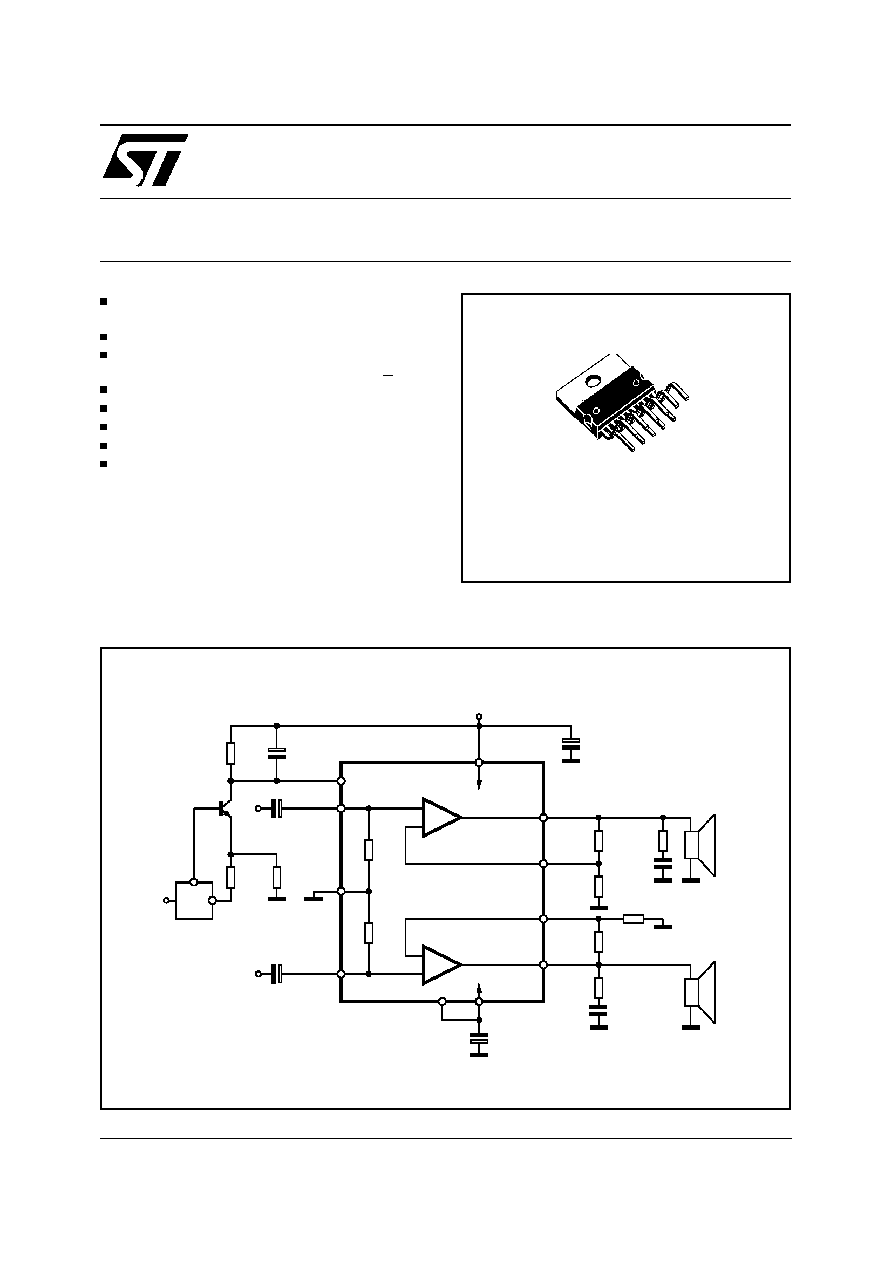TDA7265
25 +25W STEREO AMPLIFIER WITH MUTE & ST-BY
WIDE SUPPLY VOLTAGE RANGE (UP TO
±
25V ABS MAX.)
SPLIT SUPPLY
HIGH OUTPUT POWER
25 + 25W @ THD =10%, R
L
= 8
, V
S
= +20V
NO POP AT TURN-ON/OFF
MUTE (POP FREE)
STAND-BY FEATURE (LOW I
q
)
SHORT CIRCUIT PROTECTION
DESCRIPTION
The TDA7265 is class AB dual Audio power am-
plifier assembled in the Multiwatt package, spe-
cially designed for high quality sound application
as Hi-Fi music centers and stereo TV sets.
July 1998
Æ
Multiwatt11
ORDERING NUMBER: TDA7265
+5V
18K
15K
IN (L)
1
µ
F
15K
1
µ
F
MUTE/
ST-BY
GND
IN (R)
1
µ
F
4.7
4.7
100nF
100nF
OUT (L)
OUT (R)
1000
µ
F
1000
µ
F
+V
S
3
5
7
9
6
11
2
4
+
+
-
-
D94AU085
RL (L)
RL (R)
-V
S
µ
P
560
18K
IN- (L)
8
10
IN- (R)
1
560
18K
Figure 1: Typical Application Circuit
1/11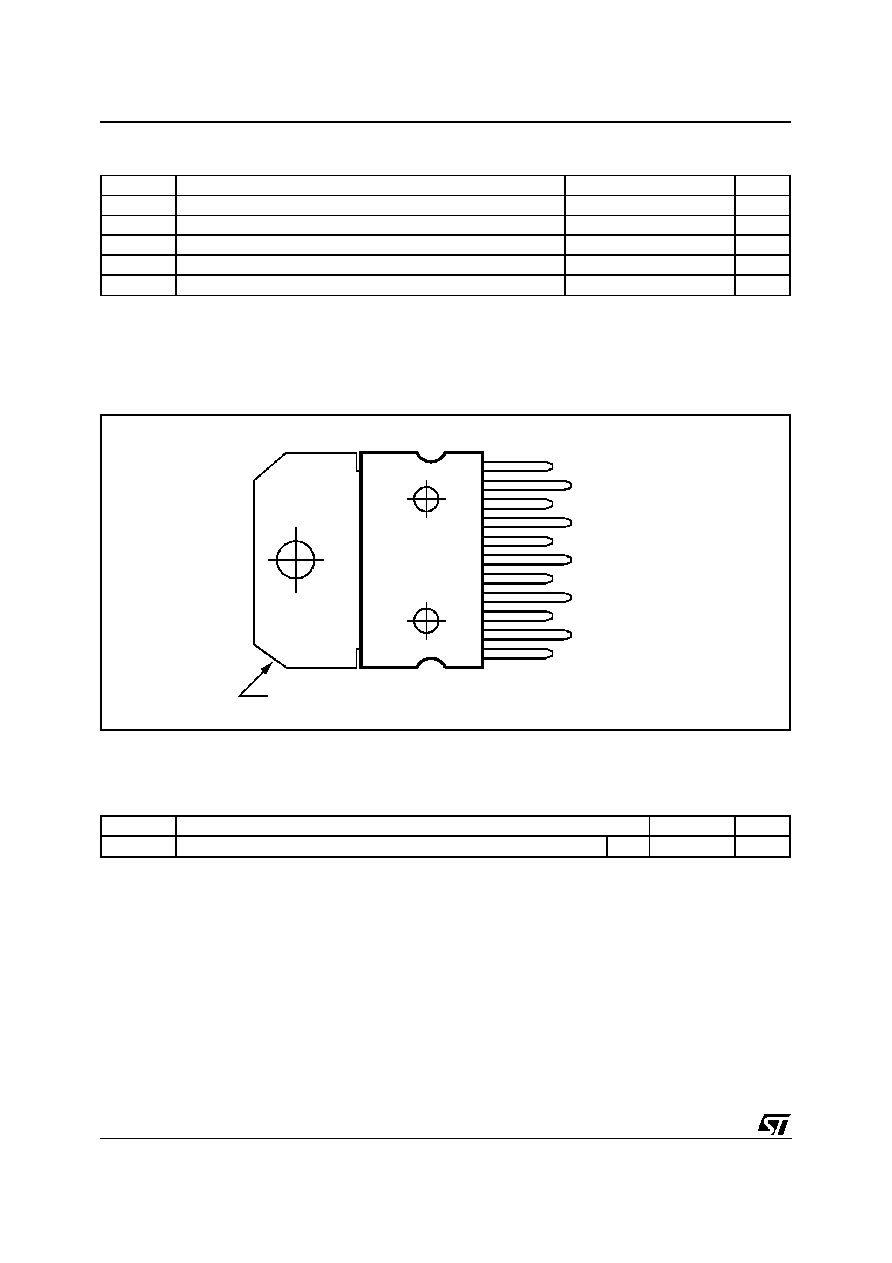ABSOLUTE MAXIMUM RATINGS
Symbol
Parameter
Value
Unit
V
S
DC Supply Voltage
±
25
V
I
O
Output Peak Current (internally limited)
4.5
A
P
tot
Power Dissipation T
case
= 70
C
30
W
T
op
Operating Temperature
-20 to 85
C
T
stg
, T
j
Storage and Junction Temperature
-40 to +150
C
1
2
3
4
5
6
7
9
10
11
8
IN+(1)
IN-(1)
GND
IN-(2)
IN+(2)
-V
S
MUTE
OUTPUT(2)
+V
S
OUTPUT(1)
-V
S
TAB CONNECTED TO PIN 6
D95AU316
PIN CONNECTION (Top view)
THERMAL DATA
Symbol
Description
Value
Unit
R
th j-case
Thermal Resistance Junction-case
Max
2
C/W
TDA7265
2/11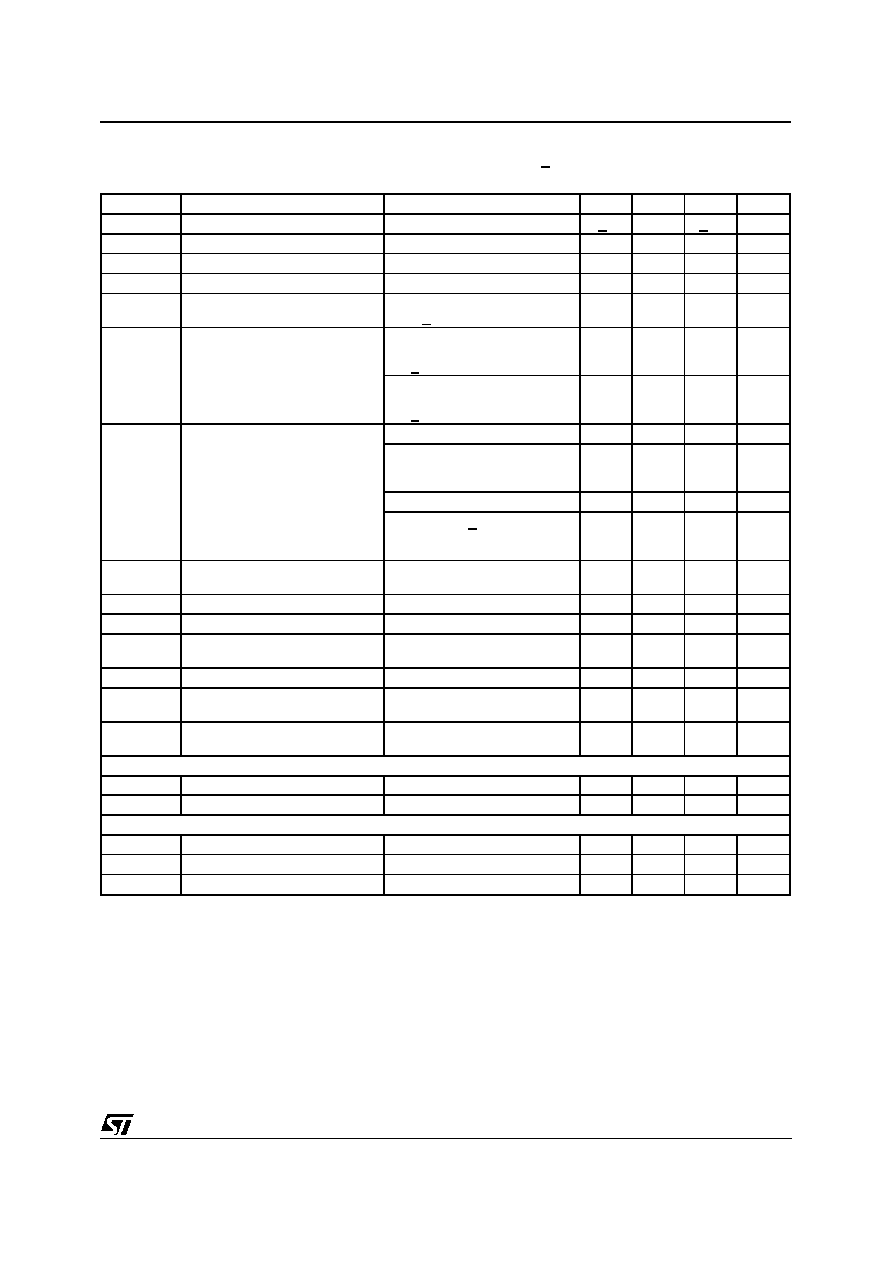ELECTRICAL CHARACTERISTICS (Refer to the test circuit, V
S
= + 20V; R
L
= 8
; R
S
= 50
;
G
V
= 30dB; f = 1KHz;
T
amb
= 25
C, unless otherwise specified.)
Symbol
Parameter
Test Condition
Min.
Typ.
Max.
Unit
V
S
Supply Range
+5
+25
V
I
q
Total Quiescent Current
80
130
mA
V
OS
Input Offset Voltage
≠20
+20
mV
I
b
Non Inverting Input Bias Current
500
nA
P
O
Music Output Power (*)
THD = 10%; R
L
= 8
;
V
S
= + 22.5V
32
W
P
O
Output Power
THD = 10%
R
L
= 8
;
V
S
+ 16V; R
L
= 4
20
25
25
W
W
THD = 1%
R
L
= 8
;
V
S
+ 16V; R
L
= 4
20
20
W
W
THD
Total Harmonic Distortion
R
L
= 8
; P
O
= 1W; f = 1KHz
0.01
%
R
L
= 8
;
P
O
= 0.1 to 15W;
f = 100Hz to 15KHz
0.7
%
R
L
= 4
; P
O
= 1W; f = 1KHz
0.02
%
R
L
= 4
; V
S
+ 16V;
P
O
= 0.1 to 12W;
f = 100Hz to 15KHz
1
%
C
T
Cross Talk
f = 1KHz
f = 10KHz
70
60
dB
dB
SR
Slew Rate
10
V/
µ
s
G
OL
Open Loop Voltage Gain
80
dB
e
N
Total Input Noise
A Curve
f = 20Hz to 22KHz
3
4
8
µ
V
µ
V
R
i
Input Resistance
15
20
K
SVR
Supply Voltage Rejection
(each channel)
fr = 100Hz
Vr = 0.5V
60
dB
T
j
Thermal Shut-down
Junction Temperature
145
C
MUTE FUNCTION [ref: +V
S
]
VT
MUTE
Mute / Play Threshold
-7
-6
-5
V
A
M
Mute Attenuation
60
70
dB
STAND-BY FUNCTION [ref: +V
S
]
VT
ST-BY
Stand-by / Mute Threshold
-3.5
-2.5
-1.5
V
A
ST-BY
Stand-by Attenuation
110
dB
I
q ST-BY
Quiescent Current @ Stand-by
3
mA
Note :
(*) FULL POWER up to. V
S
=
±
22.5V with R
L
= 8
and V
S
=
±
16V with R
L
= 4
MUSIC POWER is the maximal power which the amplifier is capable of producing across the rated load resistance (regardless of non linearity)
1 sec after the application of a sinusoidal input signal of frequency 1K Hz.
TDA7265
3/11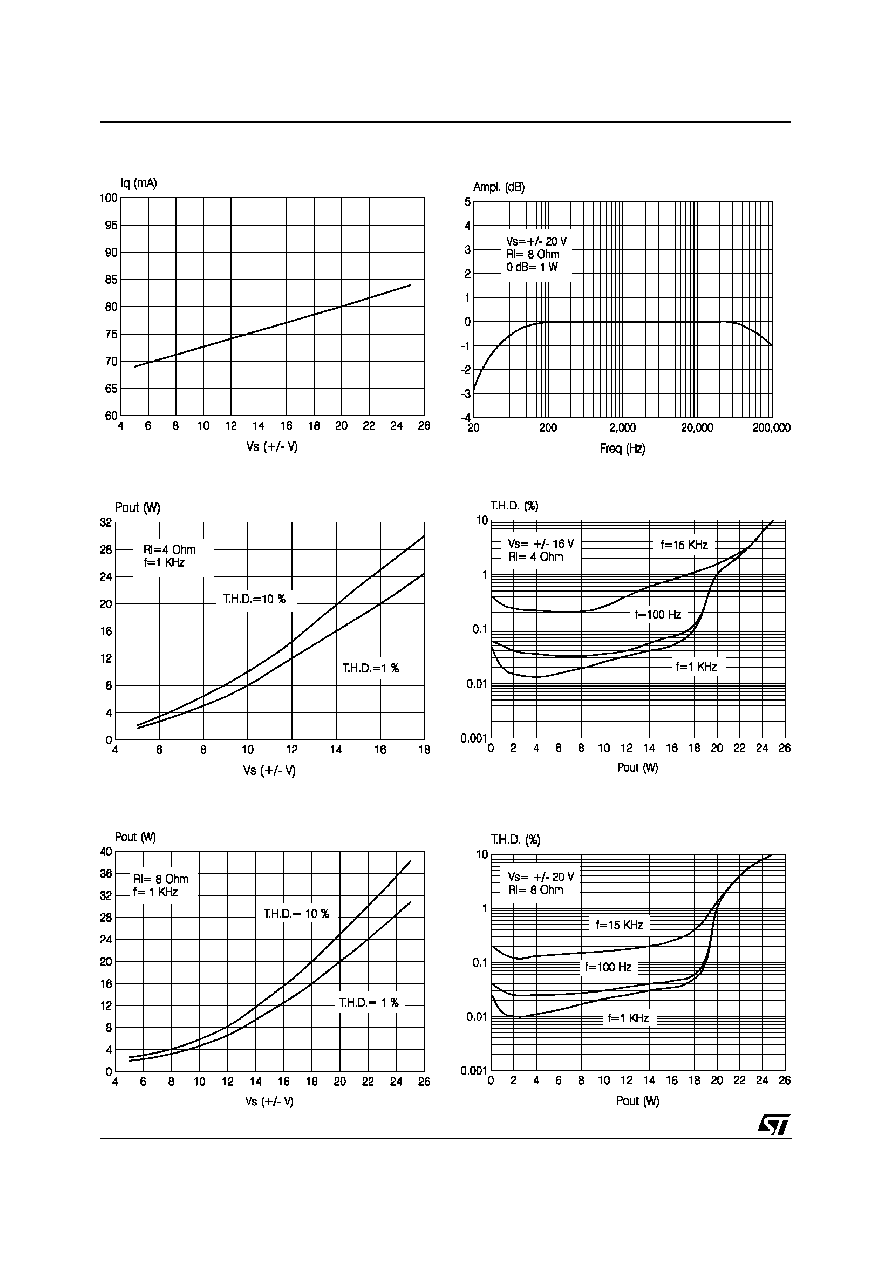Figure 2: Quiescent Current vs. Supply Voltage
Figure 3: Frequency Response
Figure 4: Output Power vs. Supply Voltage
Figure 5: T.H.D. vs. Output Power
Figure 6: Output Power vs. Supply Voltage
Figure 7: T.H.D. vs. Output Power
TDA7265
4/11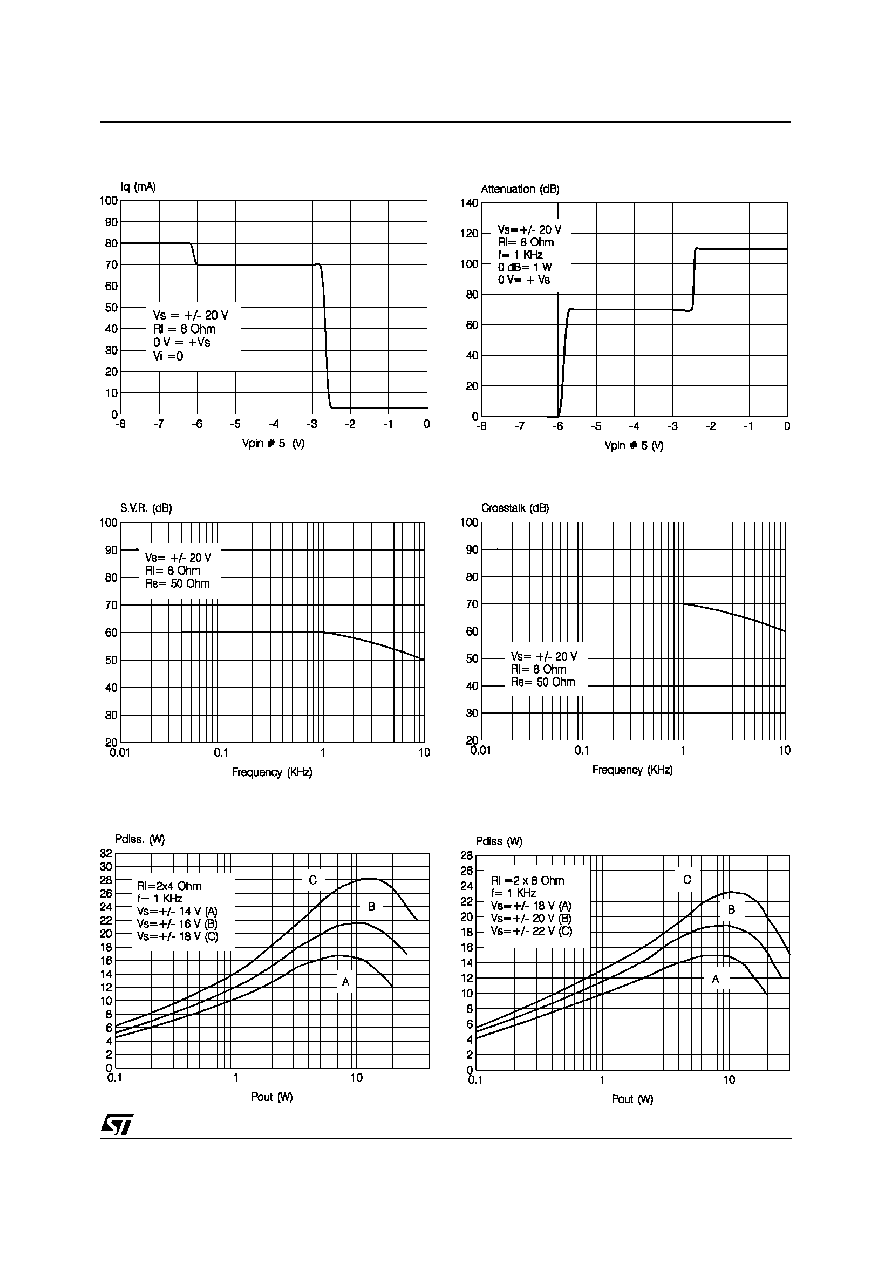Figure 8: Quiescent Current vs. Pin # 5 Voltage
Figure 9: Attenuation vs. Pin # 5 Voltage
Figure 10: SVR vs. Frequency
Figure 11: Crosstalk vs. Frequency
Figure 12: Power Dissipaton vs. Output Power
Figure 13: Power Dissipaton vs. Output Power
TDA7265
5/11